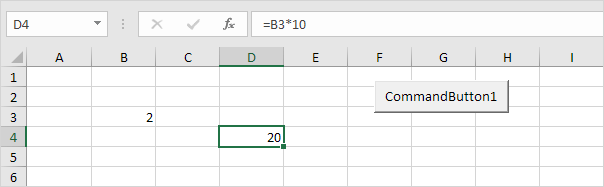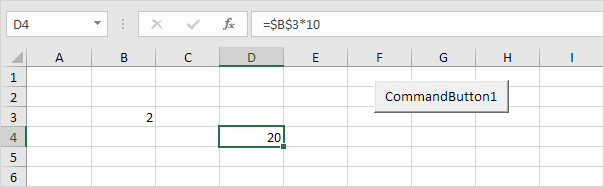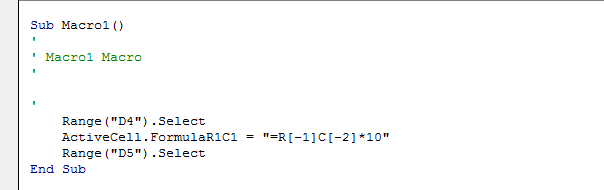# FormulaR1C1 in Excel VBA

This example illustrates the difference between A1, R1C1 and RC style in Excel VBA.

1. Place a command button on your worksheet and add the following code line (A1 style):

Range("D4").Formula = "=B3*10"

Result:2. Add the following code line (R1C1 style):

Range("D4").FormulaR1C1 = "=R3C2*10"

Result:Explanation: cell D4 references cell B3 (row 3, column 2). This is an absolute reference (\$ symbol in front of the row number and column letter).

3. Add the following code line (RC style):

Range("D4").FormulaR1C1 = "=R[-1]C[-2]*10"

Result:Explanation: cell D4 references cell B3 (one row above and 2 columns to the left). This is a relative reference. This code line gives the exact same result as the code line used at step 1.

4. Why learning about this? Because the Macro Recorder uses the FormulaR1C1 property (RC style). The Macro Recorder creates the following code lines if you enter the formula =B3*10 into cell D4.Explanation: you can see that this is the exact same code line used at step 3.

Go to Next Chapter: MsgBox